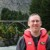# DOE – How to Design Experiments with Many Factors and Levels

Six Sigma – iSixSigma Forums General Forums Methodology DOE – How to Design Experiments with Many Factors and Levels

Viewing 7 posts - 1 through 7 (of 7 total)
• Author
Posts
• #56110Amirul Rushaidi
Participant

My title is on the Optimatization of micro EDM. I need to design experiment with 4 factors which is voltage, capacitance, machining speed and the workpiece. Each factor consists 5 levels. If I were to use full factorial, I gonna run 1024 experiments, which is quite impossible due to time constraint. I can only run about 60 experiments. So what should I do? Thank you.

0
#203110Robert Butler
Participant

What kind of a measure is “the workpiece”? My guess would be categorical unless you have some way of quantifying this variable (really small, small, medium, large, very large), (really simple, simple, average, above average, complex) etc. If it is categorical then the smallest design (we are assuming voltage, capacitance, and machining speed can be treated as continuous variables) would be a basic 3 variable saturated design (3 factors in 4 experiments) run for each of the 5 workpiece categories for a total of 20 experiments – add one replicate per group and you would have a total of 25 experiments.

If, on the other hand, workpiece can be quantified then you could run a 2 level factorial with a couple of replicates at the center point for a total of 18 experiments.

The thing to bear in mind here is that the philosophy of experimental design is that if something is going to occur it is most likely to occur at the extremes so rather than 5 levels for a continuous variable choose the minimum and maximum settings and use variations of a two level factorial design. Of course, if the one variable is categorical then you would run the smaller two level saturated design at each of the 5 categorical values.

If you are worried about the possibility of curvature then you would want to include a center point on the factorial and run your replication there. For the case of the saturated designs with the categorical variable this would bring the total number of experiments to 30 and, if all can be treated as continuous then, as previously noted, the total would be 18.

0
#203116shamshul
Guest

Naturally if we follow DMAIC, then before we do DOE at the analyze phase we should be able to see the relationship of the Y and the Xs. We should be able to see the low and high points so that we can judge whether the levels in between required in the DOE or not. If you’d studied the relationship between individual x to the y, then perhaps you can reduce the levels in the DOE and then further reduce the model to fractional design.

0
#203120Amirul Rushaidi
Participant

The workpiece parameter is based on λ·θ·ρ theory, which is the thermal conductivity (λ), melting point (θ) and electrical resistivity (ρ). So, it is numerical.

Actually this is my first time using DOE.

0
#203122Robert Butler
Participant

Then is it fair to say that it makes sense to characterize the workpiece parameter with high and low values? Which is to say – are lambda, theta, and rho connected or can they be varied independently? If it is the former then you have 4 continuous variables which means you could run a screen check in 8 points with two center points for a total of 10 experiments. If it is the latter then you actually have the situation of a 6 parameter study – lambda, theta, rho, voltage, capacitance, and machine speed. In this case you could also run a screen design in 8 experiments again with two center points for a total of 10 experiments.

An analysis of the 10 points would allow a check for significance of the factors of interest and pave the way for a design with fewer factors and the inclusion of some possible interaction terms. The analysis of the 10 point designs would also provide an initial predictive equation which could be tested to see if the observed correlations actually described possible causation.

0
#203130Chris Seider
Participant

I’d highly recommend a 2^4-1 factorial design since you are limited on experimental runs. I would use some of the runs to run multiple runs (5) at the center point you can naturally design into your experiment. Don’t forget multiple observations at each corner point of your design.

Good luck–sounds exciting.

0
#209076Andrew
Participant

You say you can run 60 experiments which is good, that gives enough data points to be confident.
The problem is that you have too many levels to get a manageable number of runs.
5 levels could be an overkill if the effect of a factor isn’t linear or quadratic.

Some options which include reducing the number of levels are:

1) 2 level Full factorial design: 16 runs to which you can add a few center-points. You may want to do more replicates as it is good to have more data points to take a decision.

2) 3 level Full factorial design: 81 runs (which is over your limit of 60) BUT if you reduce one of the factors to 2 levels and keep the other 3 at 3 levels it is only 54 runs, to which you can add a few centre-points.

0
Viewing 7 posts - 1 through 7 (of 7 total)

You must be logged in to reply to this topic.